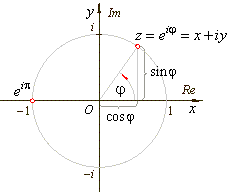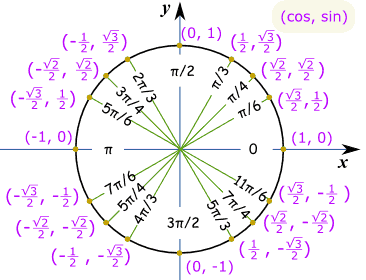# E^pi*i ?

fig 1

Given:e= cosΘ + i sinΘ (radians)

eπi=-1

Deduced

e2πi=(-1)2

e2πi=1

e(2/3)πi=11/3

e(2/3)iπ=1

e(2/3)iπ=cos(2i/3)+i sin(2i/3)

e(2/3)iπ=-1/2+i(3/2)

-1/2+i(31/2/2)=1

where n is greater than or equal to 1 or n=a/b where a is greater than or equal to 1 and b is odd1n=1

(-1/2+i(3/2))n=1

However this is absurd and I
have no idea what's wrong
(sorry about the formating it was better in docs)

#### Attachments

Last edited:

.Scott
Homework Helper
As soon as you put a denominator into the exponent, you run into trouble.
For example, ##1^1=1##. But does ##1^{1/2}=1##? It could equal -1.

Also, Look at your third "deduced" line.
Where did it come from? ##e^{(2/3)πi}=31## ?

In any case, ##e^{(2/3)πi)} = -1/2 + (\sqrt{3}/2)i##

PeroK
Homework Helper
Gold Member
2020 Award
fig 1

Given:View attachment 238257

e= cosΘ + i sinΘ (radians)

eπi=-1

Deduced

e2πi=(-1)2

e2πi=1

e(2/3)πi=31

e(2/3)iπ=1

e(2/3)iπ=cos(2i/3)+i sin(2i/3)

e(2/3)iπ=-1/2+i(3/2)

-1/2+i(31/2/2)=1

where n is greater than or equal to 1 or n=a/b where a is greater than or equal to 1 and b is oddView attachment 238258

1n=1

(-1/2+i(3/2))n=1

However this is absurd and I
have no idea what's wrong
(sorry about the formating it was better in docs)

What you have really shown is that:

##(e^{2\pi i/3})^3 = 1##

In other words:

##(\cos(2\pi /3) + i \sin(2\pi /3))^3 = 1##

And, in fact, there are three complex numbers ##z##, where ##z^3 = 1##.

But, this doesn't mean that in this case ##z = 1^{1/3} = 1##.

In the same way that ##-1 \ne \sqrt{1} = 1##, although we do have ##(-1)^2 = 1##

And, in general, we have:

##(z^n)^{1/n} \ne z##

Where ##\ne## here means is not necessarily equal to.

why does this happen?

As soon as you put a denominator into the exponent, you run into trouble.
For example, ##1^1=1##. But does ##1^{1/2}=1##? It could equal -1.

Also, Look at your third "deduced" line.
Where did it come from? ##e^{(2/3)πi}=31## ?

In any case, ##e^{(2/3)πi)} = -1/2 + (\sqrt{3}/2)i##
sorry that was meant to be one root three

PeroK
Homework Helper
Gold Member
2020 Award
why does this happen?

Why does what happen?

might this be the same reason that extraneous solutions exist?

PeroK